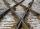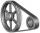# Perimeter + right triangle - math problems

1. Area of a rectangleCalculate the area of a rectangle with a diagonal of u = 12.5cm and a width of b = 3.5cm. Use the Pythagorean theorem.
2. Trapezoid MOThe rectangular trapezoid ABCD with right angle at point B, |AC| = 12, |CD| = 8, diagonals are perpendicular to each other. Calculate the perimeter and area of ​​the trapezoid.
3. GardenArea of a square garden is 6/4 of triangle garden with sides 56 m, 35 m, and 35 m. How many meters of fencing need to fence a square garden?
4. Triangle SASCalculate the area and perimeter of the triangle, if the two sides are 51 cm and 110 cm long and angle them clamped is 130 °.
5. RhombusCalculate the perimeter and area of ​​a rhombus whose diagonals are 38 cm and 55 cm long.
6. Right triangle AlefThe obvod of a right triangle is 84 cm, the hypotenuse is 37 cm long. Determine the lengths of the legs.
7. RectangleIn rectangle with sides, 6 and 3 mark the diagonal. What is the probability that a randomly selected point within the rectangle is closer to the diagonal than to any side of the rectangle?
8. Square diagonalCalculate length of the square diagonal if the perimeter is 476 cm.
9. StepsHow many steps you save if you go square estate for diagonal (crosswise), rather than circumvent on the two sides of its perimeter with 307 steps.
10. Isosceles right triangleCalculate the area of an isosceles right triangle whose perimeter is 377 cm.
11. R TrapeziumRectangular trapezium has bases 12 and 5 and area 84 cm2. What is its perimeter?
12. Rotation of the EarthCalculate the circumferential speed of the Earth's surface at a latitude of 61°​​. Consider a globe with a radius of 6378 km.
13. CapJesters hat is shaped a rotating cone. Calculate how much paper is needed to the cap 60 cm high when head circumference is 52 cm.
14. ISO triangleCalculate the area of an isosceles triangle KLM if the length of its sides are in the ratio k:l:m = 4:4:3 and has perimeter 377 mm.
15. Recursion squaresIn the square ABCD is inscribed a square so that its vertices lie at the centers of the sides of the square ABCD.The procedure of inscribing square is repeated this way. Side length of square ABCD is a = 22 cm. Calculate: a) the sum of perimeters of all
16. Circle sectionEquilateral triangle with side 33 is inscribed circle section whose center is in one of the vertices of the triangle and the arc touches the opposite side. Calculate: a) the length of the arc b) the ratio betewwn the circumference to the circle sector.
17. Right triangleCalculate the missing side b and interior angles, perimeter and area of ​​a right triangle if a=10 cm and hypotenuse c = 16 cm.
18. Track arcTwo straight tracks is in an angle 74°. They will join with circular arc with radius r=1127 m. How long will be arc connecting these lines (L)? How far is the center point of arc from track crossings (x)?
19. Perimeter and legsDetermine the perimeter of a right triangle if the length of one leg is 75% length of the second leg and its content area is 24 cm2.
20. V-beltCalculate the length of the belt on pulleys with diameters of 105 mm and 393 mm at shaft distance 697 mm.

Do you have an interesting mathematical word problem that you can't solve it? Enter it, and we can try to solve it.

To this e-mail address, we will reply solution; solved examples are also published here. Please enter the e-mail correctly and check whether you don't have a full mailbox.

Please do not submit problems from current active competitions such as Mathematical Olympiad, correspondence seminars etc...

Try perimeter of right triangle calculator. See also our right triangle calculator.